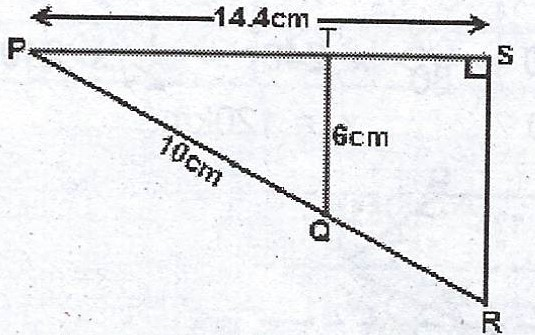### Mathematics Past Questions

3721

(a)In the diagram, < PTQ = < PSR = 90°, /PQ/ = 10 cm, /PS/ = 14.4 cm and /TQ/ = 6 cm. Calculate the area of the quadrilateral QRST.

(b) Two opposite sides of a square are each decreased by 10% while the other two are each increased by 15% to form a rectangle. Find the ratio of the area of the rectangle to that of the square.

3722

The frequency distribution of the weight of 100 participants in a high jump competition is as shown below :

 Weight (kg) 20 - 29 30 - 39 40 - 49 50 - 59 60 - 69 70 - 79 Number of  Participants 10 18 22 25 16 9

(a) Construct the cumulative frequency table.

(b) Draw the cumulative frequency curve.

(c) From the curve, estimate the : (i) median ; (ii) semi- interquartile range ; (iii) probability that a participant chosen at random weighs at least 60 kg.

3723

(a) The third term of a Geometric Progression (G.P) is 24 and its seventh term is $$4\frac{20}{27}$$. Find its first term.

(b) Given that y varies directly as x and inversely as the square of z. If y = 4, when x = 3 and z = 1, find y when x = 3 and z = 2.

3724

(a) Given that $$(\sqrt{3} - 5\sqrt{2})(\sqrt{3} + \sqrt{2}) = a + b\sqrt{6}$$, find a and b.

(b) If $$\frac{2^{1 - y} \times 2^{y - 1}}{2^{y + 2}} = 8^{2 - 3y}$$, find y.

3725

(a) If $$9 \cos x - 7 = 1$$ and $$0° \leq x \leq 90°$$, find x.

(b) Given that x is an integer, find the three greatest values of x which satisfy the inequality $$7x < 2x - 13$$.